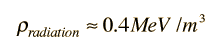# Neutrino Transparency Temperature

In evaluating the energy density in the background radiation in the universe, it is necessary to include both the photon 3K background and the neutrino background. The neutrino transparency point occurred before the photon transparency which gives us the microwave background radiation. In estimating that transparency temperature for neutrinos, Weinberg uses an entropy argument.

Presuming that the expanding universe did so under conditions of thermal equilibrium, the second law of thermodyamics suggests that entropy remained constant. The entropy per unit volume at temperature T can be approximated by

S α NTT3

where NT is the effective number of species in thermal equilibrium . This includes only those particles which have a critical temperature less than T, i.e., they can be formed from the current level of thermal energy. To keep the entropy constant for an expanding universe, the entropy must be proportional to the inverse cube of the volume so that

SR3 α NTT3R3 = constant

where R can be taken to be the separation between typical particles.

After the neutrinos in the early universe went out of equilibrium, only electrons, positrons and photons were left in thermal equilibrium, and that changed with the annihilation of the electrons and positrons at about 5 x 109K. To evaluate the effect on the temperature, one must examine the number of species which goes into the entropy expression. The effective numbers are:

 Nelectron,positron = 2 x 2 x 7/8 = 7/2 Nphoton = 2 x 1 x 1 = 2 The effective numbers for the particles are calculated as the product of the three factors: 2 if particle has distinct antiparticle, 1 if not. Number of possible orientations of the particles spin. 7/8 if particle subject to Pauli exclusion principle, 1 if not.

So with the electron-positron annihilation, the effective numbers changed.

 Nbefore = 7/2 + 2 Nafter = 2

By the constancy of entropy:

11/2 (TR)3before = 2(TR)3after

The energy release of the electron-positron annihilation changes the quantity TR by the factor:

(TRafter/TRbefore) = (11/4)1/3 = 1.401

This is the factor which allows us to calculate the effective temperature of the neutrino background. We reason that before the electron-positron annihilation, the temperature for the photons and neutrinos was the same. Since the neutrinos had decoupled at that time, the product TνR for the neutrinos remains equal to the product TeR before the annihilation. For the present universe this gives

Tν = Tphoton(4/11)1/3 = 2.725 K/1.401 = 1.9K

for the effective temperature of the neutrino background.

The amount of energy in this neutrino background can be estimated with the use of the Stefan- Boltzmann law, treating the neutrinos as massless particles at 1.9K. The ratio of the neutrino energy density in this equilibrium radiation can be expressed as a fraction of the photon energy density of about 0.25 MeV/m3

ρneutrino = ρphoton(7/4)(1.96 K/2.74 K)4 = 0.454 ρphoton = 0.11 MeV/m3

We used the fact that the neutrinos are more numerous by the factor (7/2)/2 =7/4, there being a factor of 2 for the effective number of photons because of two polarization states. The combined energy density in the background radiation is thenRelate to temperature and expansion time
Index

Reference
Weinberg
First 3 Minutes

 HyperPhysics***** Astrophysics R Nave
Go Back